Next: k-gram indexes for spelling Up: Spelling correction Previous: Forms of spelling correction   Contents   Index

## Edit distance

Given two character strings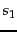and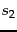, the edit distance between them is the minimum number of edit operations required to transforminto. Most commonly, the edit operations allowed for this purpose are: (i) insert a character into a string; (ii) delete a character from a string and (iii) replace a character of a string by another character; for these operations, edit distance is sometimes known as Levenshtein distance . For example, the edit distance between cat and dog is 3. In fact, the notion of edit distance can be generalized to allowing different weights for different kinds of edit operations, for instance a higher weight may be placed on replacing the character s by the character p, than on replacing it by the character a (the latter being closer to s on the keyboard). Setting weights in this way depending on the likelihood of letters substituting for each other is very effective in practice (see Section 3.4 for the separate issue of phonetic similarity). However, the remainder of our treatment here will focus on the case in which all edit operations have the same weight.

It is well-known how to compute the (weighted) edit distance between two strings in time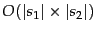, where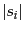denotes the length of a string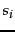. The idea is to use the dynamic programming algorithm in Figure 3.5 , where the characters inandare given in array form. The algorithm fills the (integer) entries in a matrix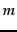whose two dimensions equal the lengths of the two strings whose edit distances is being computed; the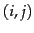entry of the matrix will hold (after the algorithm is executed) the edit distance between the strings consisting of the first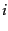characters ofand the first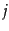characters of. The central dynamic programming step is depicted in Lines 8-10 of Figure 3.5 , where the three quantities whose minimum is taken correspond to substituting a character in, inserting a character inand inserting a character in.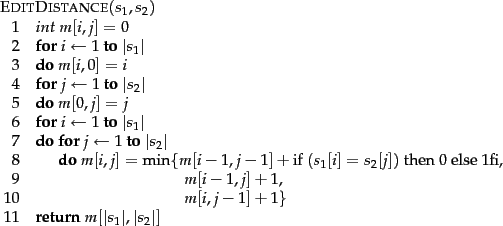Figure 3.6 shows an example Levenshtein distance computation of Figure 3.5 . The typical cell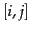has four entries formatted as a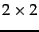cell. The lower right entry in each cell is the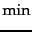of the other three, corresponding to the main dynamic programming step in Figure 3.5 . The other three entries are the three entries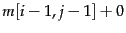or 1 depending on whether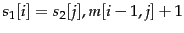and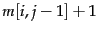. The cells with numbers in italics depict the path by which we determine the Levenshtein distance.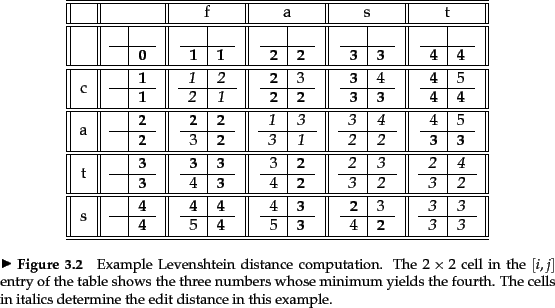The spelling correction problem however demands more than computing edit distance: given a set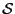of strings (corresponding to terms in the vocabulary) and a query string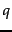, we seek the string(s) in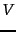of least edit distance from. We may view this as a decoding problem, in which the codewords (the strings in) are prescribed in advance. The obvious way of doing this is to compute the edit distance fromto each string in, before selecting the string(s) of minimum edit distance. This exhaustive search is inordinately expensive. Accordingly, a number of heuristics are used in practice to efficiently retrieve vocabulary terms likely to have low edit distance to the query term(s).

The simplest such heuristic is to restrict the search to dictionary terms beginning with the same letter as the query string; the hope would be that spelling errors do not occur in the first character of the query. A more sophisticated variant of this heuristic is to use a version of the permuterm index, in which we omit the end-of-word symbol \$. Consider the set of all rotations of the query string. For each rotation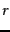from this set, we traverse the B-tree into the permuterm index, thereby retrieving all dictionary terms that have a rotation beginning with. For instance, ifis mase and we consider the rotation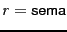, we would retrieve dictionary terms such as semantic and semaphore that do not have a small edit distance to. Unfortunately, we would miss more pertinent dictionary terms such as mare and mane. To address this, we refine this rotation scheme: for each rotation, we omit a suffix of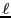characters before performing the B-tree traversal. This ensures that each term in the set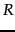of terms retrieved from the dictionary includes a long'' substring in common with. The value ofcould depend on the length of. Alternatively, we may set it to a fixed constant such as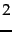.Next: k-gram indexes for spelling Up: Spelling correction Previous: Forms of spelling correction   Contents   Index